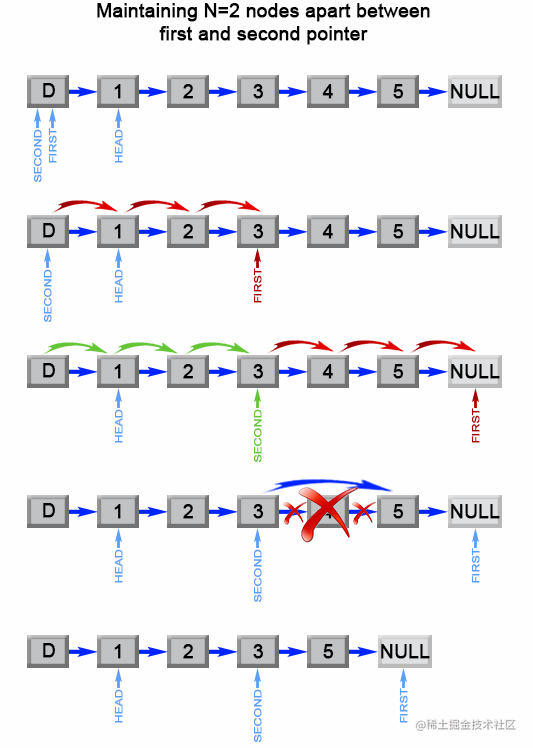# 聊一聊前端算法面试——链表和数组

## 写在前面

1. 谈一谈链表和数组的区别
2. 如何反转单向链表
3. 在数组中找出三个数，使得它们的和为N
4. 给定一个链表，删除链表的倒数第n个节点，如何实现

### 1.链表和数组的区别

1.内存组织方式不同

2.添加，删除，插入的时间复杂度不同

3.链表支持动态扩容

### 2.如何反转单向链表

``````输入: 1->2->3->4->5->NULL

``````var reverseList = function(head) {
let pre = null;
for (let cur = head; cur;) {
let nextTemp = cur.next; // 保存当前头节点的下一个节点
cur.next = pre;
pre = cur;
cur = nextTemp;
}
return pre;
};

### 3.在数组中找出三个数，使得它们的和为N

``````例如, 给定数组 nums = [-1, 0, 1, 2, -1, -4]，

[
[-1, 0, 1],
[-1, -1, 2]
]

1.对数组进行排序，如果数组的第一个元素大于0或者最后一个元素小于0，则不可能出现和为0的情况；

2.首先选定一个元素（A），再利用双指针对数组的元素进行遍历，将一个指针指向A元素的后一个元素（B），另一个指针指向数组的最后一个元素（C）；

3.判断B+C的结果是否是A的相反数，如果B+C > (-A) ,则使C指针向前移动，如果B+C <(-A) ,则使B指针向后移动；

4.如果B指针的元素与其前一个元素相等，则B指针向后移动一位；如果C指针与其后一个元素相等，则C指针向前移动一位；

5.重复以上步骤

``````var threeSum = function(nums) {
//用来存取最后结果集
let result = new Array();
//头指针
//尾指针
let end;
//固定值
let fixedVal;

//排序
nums.sort((a, b) => {
return a-b;
});

//判断数组内元素是否都为整数或负数，直接返回
if(nums > 0 || nums[nums.length - 1] < 0) return result;

// 开始遍历
for (let i = 0; i < nums.length; i++) {
//固定值
fixedVal = nums[i];
// 如果前后元素相同，跳过此次循环（固定值）
if(fixedVal === nums[i-1]) continue;
//一开始的固定值为nums,所以头指针为 i+1 下一个元素
//尾指针
end = nums.length - 1;
//如果头指针小于尾指针元素
//判断固定值+头指针+尾指针是否等于0
if(nums[head] + nums[end] + fixedVal === 0){
//声明数组，存放这三个值
let group =  new Array();
group.push(nums[end]);
group.push(fixedVal);
result.push(group);
//存放完毕之后，不要忘记头指针和尾指针的移动(否则会产生死循环)
end -= 1;
//如果头指针满足小于尾指针且移动后的指针和移动前的指针元素相等，再往前移动
}
//如果头指针满足小于尾指针且移动后的指针和移动前的指针元素相等，再往后移动
while(head < end && nums[end] === nums[end + 1]){
end -= 1;
}
//小于 0 需要移动头指针，因为尝试着让数据比原有数据大一点
}else if(nums[head] + nums[end] + fixedVal < 0){
}else{
//否则，尾指针向前移动，让数据小于元数据
end--;
}
}
}
return result;
};

### 4.给定一个链表，删除链表的倒数第n个节点，如何实现

``````给定一个链表: 1->2->3->4->5, 和 n = 2.``````var removeNthFromEnd = function(head, n) {
let first = head; // 慢指针
for (let i = 0; i < n; i++) {
first = first.next;
}
if (!first) return head.next; // 当链表长度为n时，删除第一个节点

let second = head; // 快指针
while (first.next) {
first = first.next;
second = second.next;
}
second.next = second.next.next;